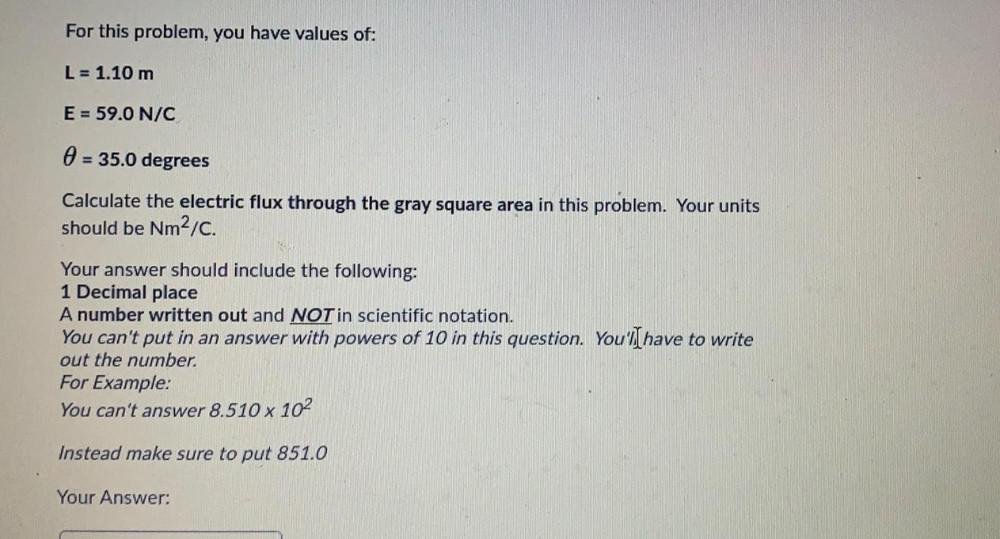Question:

# For this problem, you have values of: L = 1.10 m E = 59.0 N/C 0 = 35.0 degrees Calculate the electric flux through the gray squaFor this problem, you have values of: L = 1.10 m E = 59.0 N/C 0 = 35.0 degrees Calculate the electric flux through the gray square area in this problem. Your units should be Nm2/C. Your answer should include the following: 1 Decimal place A number written out and NOT in scientific notation. You can't put in an answer with powers of 10 in this question. You'lI have to write out the number For Example: You can't answer 8.510 x 102 Instead make sure to put 851.0 Your Answer: